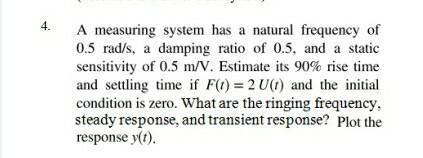# Question Solved1 Answer4. A measuring system has a natural frequency of 0.5 rad/s, a damping ratio of 0.5, and a static sensitivity of 0.5 m/V. Estimate its 90% rise time and settling time if F(t) = 2 U(1) and the initial condition is zero. What are the ringing frequency steady response, and transient response? Plot the response y(t).SFTWNY The Asker · Mechanical EngineeringTranscribed Image Text: 4. A measuring system has a natural frequency of 0.5 rad/s, a damping ratio of 0.5, and a static sensitivity of 0.5 m/V. Estimate its 90% rise time and settling time if F(t) = 2 U(1) and the initial condition is zero. What are the ringing frequency steady response, and transient response? Plot the response y(t).
More
Transcribed Image Text: 4. A measuring system has a natural frequency of 0.5 rad/s, a damping ratio of 0.5, and a static sensitivity of 0.5 m/V. Estimate its 90% rise time and settling time if F(t) = 2 U(1) and the initial condition is zero. What are the ringing frequency steady response, and transient response? Plot the response y(t).